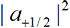Principles and Concepts
of Quantum Physics
Implications of
Quantum Physics

13a. Details of Small Parts.

Summary
In contrast to the classical case, a small part of the wave function of quantum physics carries the full mass, energy, momentum, spin and charge. Details.

We will illustrate here the idea that a small part of a wave function carries the full energy and momentum, but the argument would be similar for the other properties (spin, charge, mass) and for any kind of ‘particle.’

We shoot a spinparticle into a Stern-Gerlach apparatus. Before the particle reaches the magnet, the wave function is(13A-1)
whereis sharply peaked about k0. Then to a good approximation,(13A-2)
so that the initial wave function is essentially an eigenfunction of energy and momentum.
Now the wave function goes through the magnet and splits into two parts,(13A-3)
where the primed and double-primed k’s indicate where the corresponding f’s have a sharp peak, with. But we see that(13A-4)
So each of the two separate parts has (nearly) the same energy and momentum as the original wave. The energy and momentum did not split up according to the values ofand. This means the small part of the light wave function that hits one electron in the photoelectric (or Compton) effect carries the full energy and momentum (and spin) of the wave function.

To put the argument another way, mass, energy, momentum, spin, and charge are independent of the norm (size) of the wave function. So each small part (having a small norm) carries the same amount of these quantities as it would if the norm were 1.